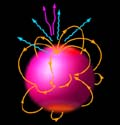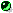Analytical EOSs of Neutron Star Matter
Fitting programs

 These analytical representations are designed for EOSs FPS, SLy4, and APR (APR is available only in the Fortran code, there is no table or C/C++ code). For "Brussels-Montreal" EOSs BSk19-22 and BSk24-26, follow this link. For other EOSs, see this collection of links.
Neutron Star Group

Description

The equations of state (EOSs) for neutron-star matter are fitted from mass density rho about 105 g cm-3 to a few times 1015 g cm-3 (baryon number density n about 10-10 fm-3 - a few fm-3). At the low end of this density range, the EOS starts to depend on temperature T. The fits are extended below this density by matching to the dependence P=3.5x1014rho, where pressure P and rho are in CGS units; this is an approximation for the EOS of iron at T = 107 K and rho = (0.1 - 1000) g cm-3.

Arguments of the program:

• MODE - an integer (1, 2, or 3) that identifies which of the arguments is the input (XN, RHO, or H1)
• KEOS - an integer (1, 2, or 3) that identifies which EOS has to be used (SLy4, FPS, or SLy4+APR*)
• XN - number density n in fm-3
• RHO - mass density rho in g cm-3;
RLG=log10(RHO)
• H1 - h/h0-1, where h is the enthalpy per baryon, and h0=m0c2 is its value near the surface
• P - pressure P in dyn cm-2;
PLG=log10(P)
• Gamma - adiabatic index, (d log P) / (d log n)
(for more explanations and definitions see the references).

The subroutine NSEOSFIT(MODE,KEOS,XN,RHO,H1,P,Gamma) returns 4 fitted arguments for 1 input (XN, RHO, or H1). It uses subroutines that realize separate fits: P(rho), n(rho), rho(n), rho(H1). Typical accuracy of the fits is a few percent or better. To achieve the perfect thermodynamic consistency, however, it is advised not to use all the fitted outputs, but to calculate either XN(RHO,P) or RHO(XN,P) using the thermodynamic identities given, e.g., by Eqs.(2),(3) of the basic reference paper (Haensel and Potekhin 2004).SLyFPSFortranC/C++